## 5.3.2 Coherent Reversal Model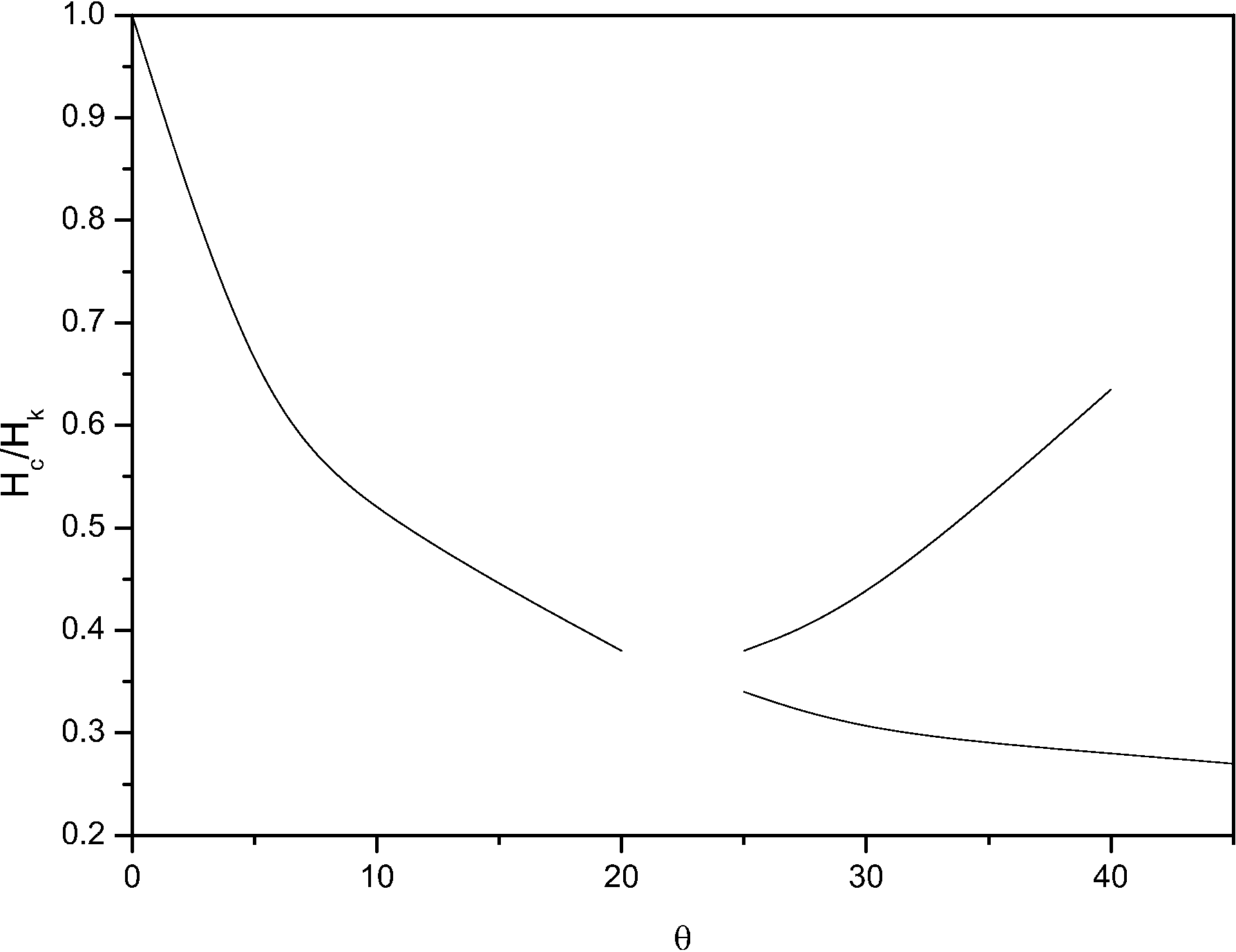As a first approach we can use a simple model of an Fe film to compare with the results in the lithographed film. This simple model is equivalent to the Stoner-Wohlfarth model for uniaxial anisotropy particles, replacing the uniaxial anisotropy with the Fe twofold anisotropy. We consider only in-plane magnetization and do not allow in-plane magnetization variations, so that coherent reversal is supposed. As a consequence the energy can be expressed as a function of a single coordinate, the angle between the magnetization and the applied field direction: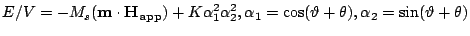(5.1)

where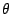is the angle between the easy axis and the applied field direction. The magnetization will evolve through a reversible rotation, until the state of the magnetization becomes an inflexion point of the energy function and another solution branch becomes the minimum. We can only find analytic solution in the case of applied field in the direction of the easy axis or the hard axis. For an easy or hard axis loop the energy derivative becomes: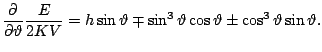(5.2)

The hysteresis loop can be calculated verifying the stability of the zeroes of Eq. (5.2). The rest of the cases have to be solved numerically.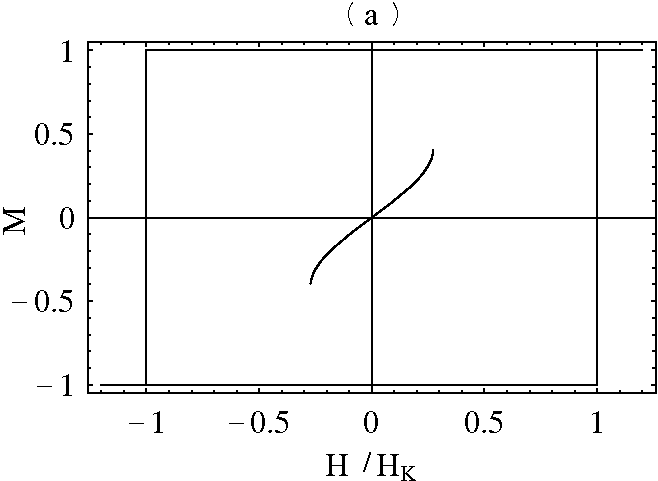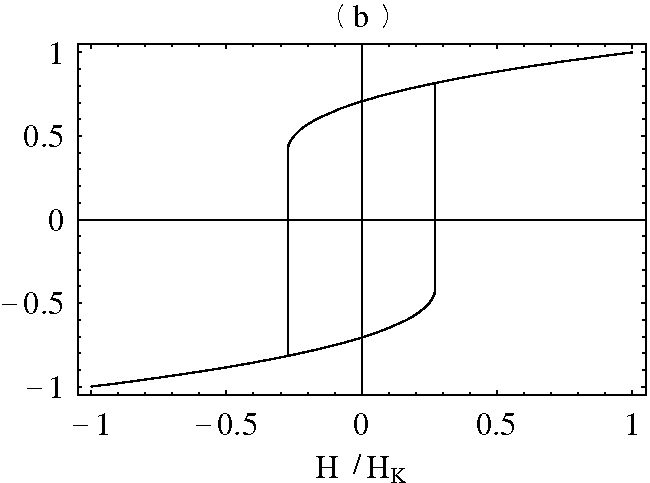In Fig. 5.14 we show the dependence of the magnetization jumps on the angle. The model predicts two jumps for angles larger than a critical value, except for the hard axis configuration in which only one jump appears. The existence of two jumps is the consequence of the biaxial anisotropy. When the minimum opposite to the field direction becomes unstable an intermediate minimum still exists that does not correspond to the alignment with field. In fact the system finds the next relative minimum along the anglebut not the absolute minimum. The second jump will occur when this intermediate minimum becomes also unstable. In Fig. 5.15(a) the alternative minimum is shown for an easy axis loop. This minimum does not exist when the branch corresponding to the magnetization saturated along the positive direction is unstable and, therefore, the magnetization will never be in such state during hysteresis processes. The model predicts the occurrence of two coercive fields, observed experimentally. However, in the experimental situation such fields existed for all applied field angles. Clearly, the model does not include the possibility of inhomogeneous magnetization reversal.

2008-04-04